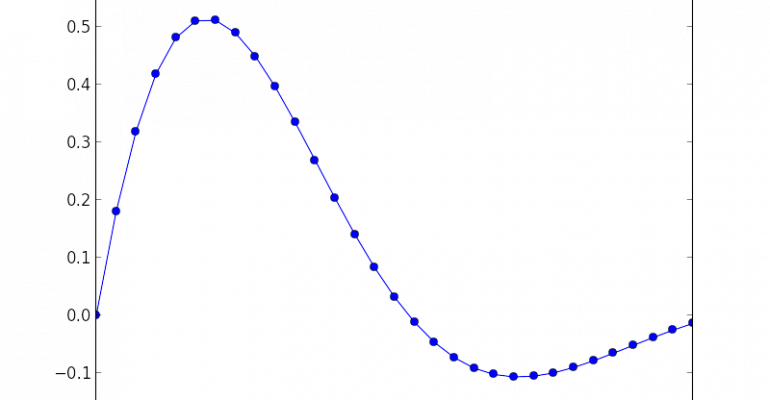Thanks to theidioms.com

### Infosys Tech Scholars Scholarship## Matplotlib savefig method – Save plots and figures in Python# Matplotlib savefig method – Save plots and figures in Python

Greetings! Some links on this site are affiliate links. That means that, if you choose to make a purchase, The Click Reader may earn a small commission at no extra cost to you. We greatly appreciate your support!

The Matplotlib savefig method is used to save Matplotib figures in Python.

## Syntax for Matplotlib savefig

The syntax for Matplotlib savefig() is as follows:

```savefig(fname, dpi=None, facecolor='w', edgecolor='w',
orientation='portrait', papertype=None, format=None,

Some important parameters of savefig is as follows:

• fname: str or path-like or binary file-like

A path, or a Python file-like object, or possibly some backend-dependent object such as `matplotlib.backends.backend_pdf.PdfPages`.

If format is set, it determines the output format, and the file is saved as fname. Note that fname is used verbatim, and there is no attempt to make the extension, if any, of fname match format, and no extension is appended.

If format is not set, then the format is inferred from the extension of fname, if there is one. If format is not set and fname has no extension, then the file is saved with `rcParams["savefig.format"]` (default: `'png'`) and the appropriate extension is appended to fname.

• dpi: float or ‘figure’, default: `rcParams["savefig.dpi"]` (default: `'figure'`)

The resolution in dots per inch. If ‘figure’, use the figure’s dpi value.

## Usage of Matplotlib savefig

The Matplotlib savefig method can be used as shown in the example below:

```import matplotlib.pyplot as plt
import numpy as np

# Sample data points
x = np.linspace(0,6,31)
y = np.exp(-0.5*x) * np.sin(x)

# Plotting
plt.plot(x, y, 'bo-')

# Saving the figure
plt.savefig('figure.png', dpi = 1200)```

This is how you save a plot in Matplotlib using Matplotlib’s savefig method.Do you want to learn Python, Data Science, and Machine Learning while getting certified? Here are some best selling Datacamp courses that we recommend you enroll in:

1. Introduction to Python (Free Course) - 1,000,000+ students already enrolled!
2. Introduction to Data Science  in Python- 400,000+ students already enrolled!
3. Introduction to TensorFlow for Deep Learning with Python - 90,000+ students already enrolled!
4. Data Science and Machine Learning Bootcamp with R - 70,000+ students already enrolled!